# Part 1: Preliminary

• True Positives (TP, blue distribution) are the people that truly have the COVID-19 virus.
• True Negatives (TN, red distribution) are the people that truly DO NOT have the COVID-19 virus.
• False Positives (FP) are the people that are truly NOT sick but based on the test, they were falsely (False) denoted as sick (Positives).
• False Negatives (FN) are the people that are truly sick but based on the test, they were falsely (False) denoted as NOT sick (Negative).

For the perfect case, we would want high values TP and TN and zero FP and FN — this would be the perfect model with the perfect ROC curve.

# Part 2: ROC

A receiver operating characteristic curve (ROC) curve is a plot that shows the diagnostic ability of a binary classifier as its discrimination threshold is varied.

The ROC curve is created by plotting the true positive rate (TPR) against the false positive rate (FPR) at various threshold settings. In other words, the ROC curve shows the trade-off of TPR and FPR for different threshold settings of the underlying model.

If the curve is above the diagonal, the model is good and above chance (chance is 50% for a binary case). If the curve is below the diagonal, the model is bad

The AUC (area under the curve) indicates if the curve is above or below the diagonal (chance level). AUC ranges in value from 0 to 1. A model whose predictions are 100% wrong has an AUC of 0.0 and one whose predictions are 100% correct has an AUC of 1.0.

The True Positive Rate and the False Positive Rate are just 2 scalars. How can we really have a curve in the ROC plot?

This is achieved by varying some threshold settings. The ROC curve shows the trade-off of TPR and FPR for different thresholds.

For instance, in the case of a Support Vector Machine (SVC) this threshold is nothing more that the bias term in the decision boundary equation. So, we would vary this bias (this would change the position of the decision boundary) and estimate the FPR and TPR for the given values of the bias.

The ROC curve is only defined for binary classification problems. However, there is a way to integrate it into multi-class classification problems. To do so, if we have N classes then we will need to define several models.

For example, if we have N=3 classes then we will need to define the following cases: case/model 1 for class 1 vs class 2, case/model 2 for class 1 vs class 2, and case/model 3 for class 1 vs class 3.

Remember that in our Covid-19 test example, we had 2 possible outcomes i.e. affected by the virus (Positives) and not affected (Negatives). Similarly, in the multi-class cases, we again have to define the Positive and Negative outcomes.

In the multi-class case, for each case the positive class is the second one:

* for case 1: “class 1 vs class 2”, the positive class is class 2

* for case 2: “class 2 vs class 3”, the positive class is class 3

* for case 3: “class 1 vs class 3”, the positive class is class 3

In other words, we can think of this as follows: We ask the classifier “Is this sample Positive or Negative?” and the classifier will predict the label (positive or negative). The ROC will be estimated for each case 1,2,3 independently.

# Reference

--

--

--

Love podcasts or audiobooks? Learn on the go with our new app.

## Making monolingual sentence embeddings multilingual using knowledge distillation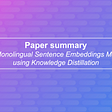## My First Work With PyTorch## Week 3: Always shoot your shot## Write an Algorithm for a Dog Identification App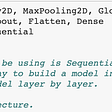## Transfer Learning for Text Classification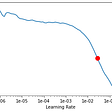## Learn linear regression with simple example.## [Lecture Notes] Loss is a bad thing. Minimize it.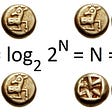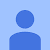## Using Machine Learning to predict Customer Churn## ML Model lifecycle management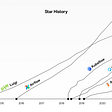## RETRIEVE: Coreset Selection for Efficient and Robust Semi-Supervised Learning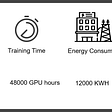## Short Term Predictions of Traffic Flow Characteristics using ML Techniques